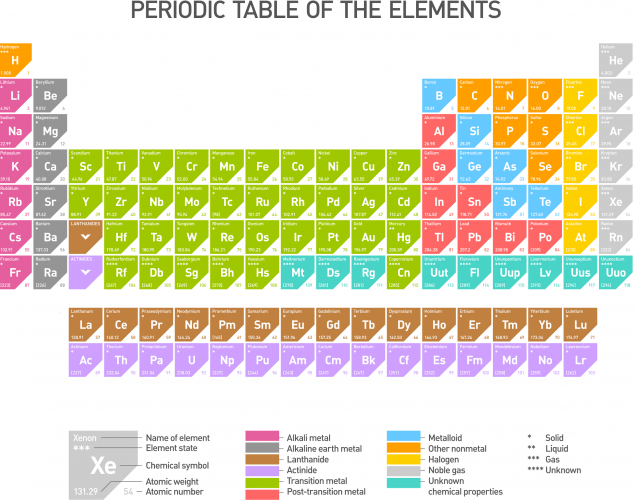• ## Accessibility

Open the accessibility toolbar to change fonts and contrast, access a dictionary, use a ruler and more

This is the Periodic Table of the elements (click on it to see a larger version):Chemical symbols are used to show an element instead of using the element name. For example, oxygen is shown as O and calcium is shown as Ca. Some elements have one letter symbols, whereas some others have two letter symbols. It is imperative that the first letter of each symbol is a capital letter to avoid confusion. Check the following: Co is the symbol for cobalt. Writing it as CO would make it stand for carbon monoxide, so there would be confusion in interpreting the symbol.

Chemical formulae

An atom is a stable chemical entity, a whole. An atom cannot be broken down by any chemical process. It is the smallest entity of an element that can be found in nature. Some elements can stand as atoms alone, whereas some others need to form molecules – two atoms together. A molecule refers to two or more atoms that have chemically bonded to make a stable entity. For example, sodium (Na) is found in nature as one atom. Oxygen, on the other hand, cannot stand alone as one atom (O). The air we breathe in contains molecules of oxygen, i.e. two oxygen atoms bonded together. We refer to a molecule of oxygen as O2, where O shows that the element is oxygen and the subscript 2 shows we are referring to two oxygen atoms, which we have come to recognize as a molecule of oxygen. Note that it would be wrong to write O2. The number must always be at the bottom after the element that it refers to.

Elements of the periodic table react and form compounds. We use chemical formulae to refer to those compounds. Formulae is the plural of the word 'formula'. There are rules to follow when naming those compounds. The formula must show the atoms that the chemical compound contains. For example, carbon dioxide contains one carbon atom and two oxygen atoms. We write this as CO2. This clearly reflects the one carbon atom and the two oxygen atoms. As for element molecules above, CO2 would be wrong.

Chemical formulae look more complicated for complex compounds. Potassium nitrate's formula is KNO3. Do not worry, but break it down slowly. K is the symbol for potassium (you may have to search the periodic table until you learn the main symbols). There is no number below it, so there is only one potassium atom in potassium nitrate. N is the symbol for nitrogen and no numbers below means there is only one nitrogen atom. Finally, O is the symbol for oxygen. Number 3 is below it, so three oxygen atoms are found in potassium nitrate.

Chemical formulae can get a bit harder but no harder than the following example. Your maths knowledge would be beneficial here. Aluminium sulfate has a more complex formula, Al2(SO4)3. Aluminium sulfate contains two aluminium atoms and then the group SO4 three times. Anything inside the brackets must be multiplied by number 3, because this is the subscript that refers to the whole bracket. Here we go: 3 times S gives 3 sulfur atoms, and 3 times O4 gives 3 × 4 = 12 oxygen atoms.

Now we need to look at the rules for naming the compounds from looking at the formula:

Rule 1: Naming simple compounds

Compounds that are formed by two elements, a metal and a non-metal, are named by the name of the metal (the first symbol of the formula) followed by the name of the non-metal with the ending -ide. Let's look at some examples. NaCl is made of sodium (Na) and chlorine (Cl). Its name would be the name of the metal (sodium) followed by the name of the non-metal (chlorine) with -ide at the end; sodium chloride.

Have a go at naming the following compound, FeS, Fe is iron and S is sulfur.

Did you answer iron sulfide? Correct!

Rule 2: Naming further compounds

Some compounds may contain a metal, a non-metal and oxygen, like Al2(SO4)3 above. In this case, we follow rule 1, but change the ending to -ate. Aluminium is the metal, sulfur is the non-metal, so it becomes aluminium sulfate. Another example is Al(BrO3)3. Its name would be aluminium bromate.

10 questions